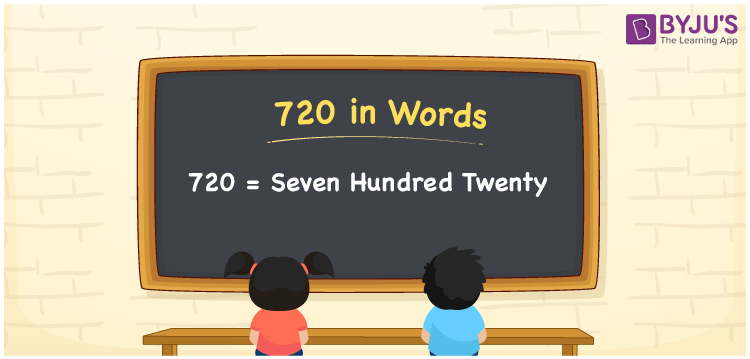# 720 in Words

720 in words can be written as Seven Hundred Twenty. The concepts like counting numbers which are important from primary education are discussed here in brief. For instance, if you spend Rs. 720 in buying shoes, then you can say that “I bought shoes for Seven Hundred Twenty Rupees”. The English alphabet is used to write 720 in words. Students can get a clear knowledge of numbers in words with the help of the study materials given at BYJU’S. The number 720 can be read as “Seven Hundred Twenty” in English.

 720 in words Seven Hundred Twenty Seven Hundred Twenty in Numbers 720

## 720 in English Words## How to Write 720 in Words?

Place value chart and expanded form of 720 can be learnt easily in this section. There are three digits in the number 720. The place value chart for 720 is provided below for reference to help students understand the concepts effectively.

 Hundreds Tens Ones 7 2 0

The expanded form of 720 is provided below:

7 × Hundred + 2 × Ten + 0 × One

= 2 × 100 + 2 × 10 + 0 × 1

= 720

= Seven Hundred Twenty

Hence, 720 in words is written as Seven Hundred Twenty.

720 is a natural number that precedes 721 and succeeds 719.

720 in words – Seven Hundred Twenty

Is 720 an odd number? – No

Is 720 an even number? – Yes

Is 720 a perfect square number? – No

Is 720 a perfect cube number? – No

Is 720 a prime number? – No

Is 720 a composite number? – Yes

## Frequently Asked Questions on 720 in Words

Q1

### How can 720 be written in words?

720 can be written as “Seven Hundred Twenty” in words.
Q2

### Is 720 an odd number?

No, 720 is not an odd number because it is divisible by 2.
720/2 = 360
Q3

### Write Seven Hundred Twenty in numbers.

Seven Hundred Twenty can be written in numbers as 720.